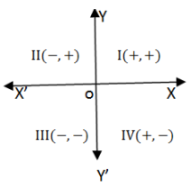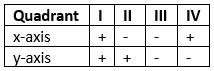Courses

# Test: Coordinate Geometry- 1

## 10 Questions MCQ Test Quantitative Aptitude (Quant) | Test: Coordinate Geometry- 1

Description
This mock test of Test: Coordinate Geometry- 1 for Quant helps you for every Quant entrance exam. This contains 10 Multiple Choice Questions for Quant Test: Coordinate Geometry- 1 (mcq) to study with solutions a complete question bank. The solved questions answers in this Test: Coordinate Geometry- 1 quiz give you a good mix of easy questions and tough questions. Quant students definitely take this Test: Coordinate Geometry- 1 exercise for a better result in the exam. You can find other Test: Coordinate Geometry- 1 extra questions, long questions & short questions for Quant on EduRev as well by searching above.
QUESTION: 1

### Q.  We divided the plane of the paper into four equal parts. by drawing two mutually perpendicular lines, X'OX and YOY'. These lines are called the axes. Here X'OX is called x-axis and YOY' is called y-axis. There axes divide the plane of the paper into four parts, called quadrants.The position of a point in a plane is denoted by an ordered pair (a,b), where a is called the x co-ordinate and y is called y co-ordinate.In which quadrant does the point(-4, -7) lie?

Solution:

The point (-4, -7) lies in 3rd quadrant.

QUESTION: 2

### In which quadrant does the point(1, 5) lie?

Solution:

The point (1, 5) lies in 1st quadrant.

QUESTION: 3

### In which quadrant does the point(9, -2) lie?

Solution:

The point (9, -2) lies in 4th quadrant.

QUESTION: 4

In which quadrant does the point(-7, 6) lie?

Solution:

The point (-7, 6) lies in 2nd quadrant.

QUESTION: 5

In which quadrant does the point(0, 9) lie?

Solution:

The point (0, 9) lies in y-axis.

QUESTION: 6

In which quadrant does the point(9, 0) lie?

Solution:

The point (9, 0) lies in x-axis.

QUESTION: 7

Find the coordinates of the point equidistant from the points A(1, 2), B(3, –4) and C(5, –6).

Solution:

Given three points A(1,2) B(3,-4) and C(5,-6).

To find the perpendicular bisectors of AB:-

• The slope of AB:
= -4-2 / 3-1
= -3
• The slope of line perpendicular to AB:
= 1 / 3
• The mid-point of AB is:
= (1 + 3 / 2, 2 - 4 / 2)
= (2, -1)
• The equation of perpendicular line is:
y = 1 / 3(x - 2) - 1

To find the perpendicular bisectors of AC:-

• The slope of AC:
= -6 - 2 / 5 - 1
= -2
• The slope of line perpendicular to AC:
= 1 /2
• The mid-point of AC is:
= (1 + 5 / 2, 2-6 / 2)
(3, -2)
• The equation of perpendicular line is:
y = 1 / 2(x - 3) - 2

Now, solve the above two equations:-

⇒ 1 / 3(x - 2) - 1 = 1 / 2(x - 3) - 2
⇒ 2(x - 2) - 6 = 3(x - 3) - 12
⇒ x = 11
⇒ y = 1 / 2(x - 3) - 2 = 1 / 2(11 - 3) - 2 = 2

The coordinates of the points equidistant from the point A(1, 2), B(3, -4) and C(5, -6) are (11, 2)

QUESTION: 8

Find the distance of the point A(3, -3) from the origin.

Solution:

OA = √32+(-3)2 = √9+9 = √18 = 3√2

QUESTION: 9

P is a point on x-axis at a distance of 4 units from y-axis to its right. The co-ordinates of P are:

Solution:

The co-ordinates of P are A(4, 0)

QUESTION: 10

A is a point on y-axis at a distance of 5 units from x-axis lying below x-axis. The co-ordinates of A are:

Solution:

The co-ordinates of A are A(0, -5)